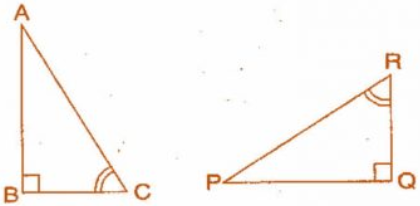Q

# If Delta ABC and Delta PQR are to be congruent, name one additional pair of corresponding parts. What criterion did you use?

9. If  and  are to be congruent, name one additional pair of corresponding parts. What criterion did you use?Views

Given

One additional pair which is not given in the figure is

We used the ASA Criterion as the two corresponding angles are given and we figured out the side by congruency.

Exams
Articles
Questions